# Fimd the market price and demand for the pair of supply and demand equAtions: supply: Q=88p+653 Demand: q=1903- 37 p

Fimd the market price and demand for the pair of supply and demand equAtions:

supply: Q=88p+653
Demand: q=1903- 37 p

When supply = demand, Q = q
88 p + 653 = 1903 - 37p
125 p = 1250
p = 10
demand = 1903 - 370 = 1533 units

The supply equation looks rather phony. Why supply any at zero price?
##### Add Answer of: Fimd the market price and demand for the pair of supply and demand equAtions: supply: Q=88p+653 Demand: q=1903- 37 p
Similar Homework Help Questions
• ### The next 3 questions involve the following supply and demand equations Supply: q(1/4)P Demand: q 6-...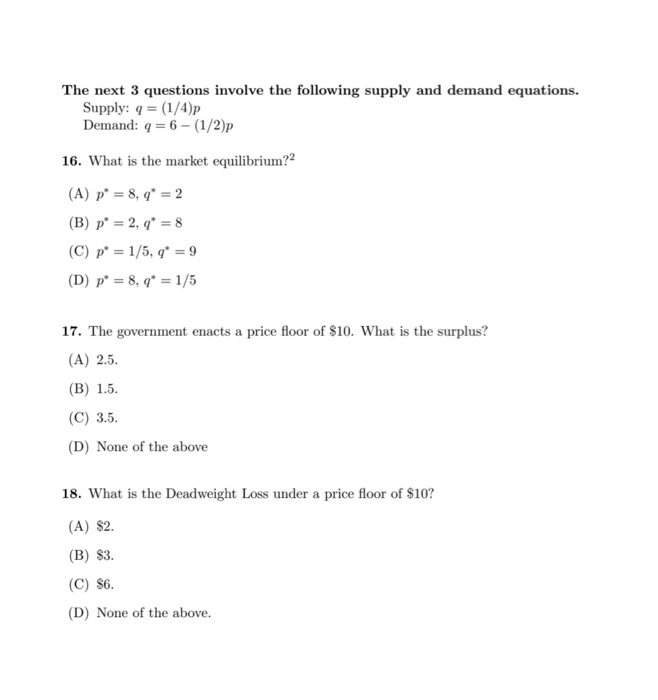The next 3 questions involve the following supply and demand equations Supply: q(1/4)P Demand: q 6- (1/2)p 16. What is the market equilibrium?2 (A) p 8, q2 (B) p 2, q8 (C) p 1/5, q = 9 (D) p 8, q = 1/5 17. The government enacts a price floor of \$10. What is the surplus? (A) 2.5 (В) 1.5. (C) 3.5 (D) None of the above 18. What is the Deadweight Loss under a price floor of \$10? (A)...

• ### Find the price and quantity that will satisfy the condition fpr static efficiency in the use of water resources given the following demand and supply equations for water: Demand: P=600-Q Supply: P=2+Q

Find the price and quantity that will satisfy the condition fpr static efficiency in the use of water resources given the following demand and supply equations for water: Demand: P=600-Q Supply: P=2+Q

• ### part b Suppose that a market is described by the following supply and demand equations Q...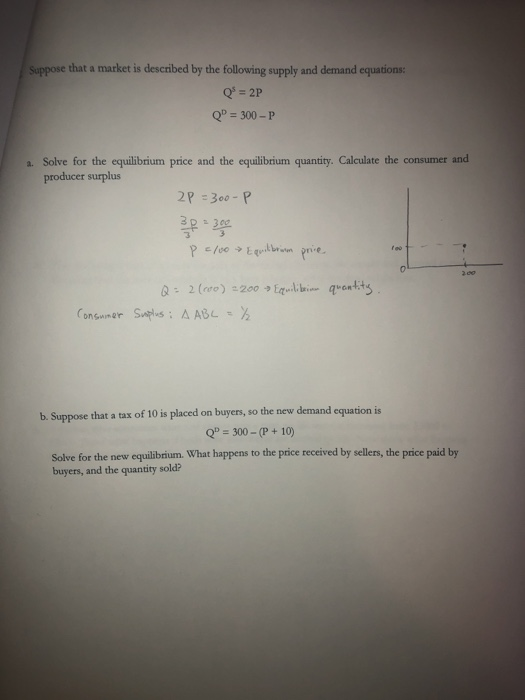part b Suppose that a market is described by the following supply and demand equations Q = 2P QP = 300-P a. Solve for the equilibrium price and the equilibrium quantity. Calculate the consumer and producer surplus 2P =300-P P 2100 Equilibrium price quantity Q = 2(000) =200 Equilibri Suplus: A ABL = ²2 Consumer b. Suppose that a tax of 10 is placed on buyers, so the new demand equation is Q" = 300 - (P+10) Solve for the...

• ### The market demand and supply is described by the following equations: Q = 100 - P Q=2P - 20 1) Find the market equilibr...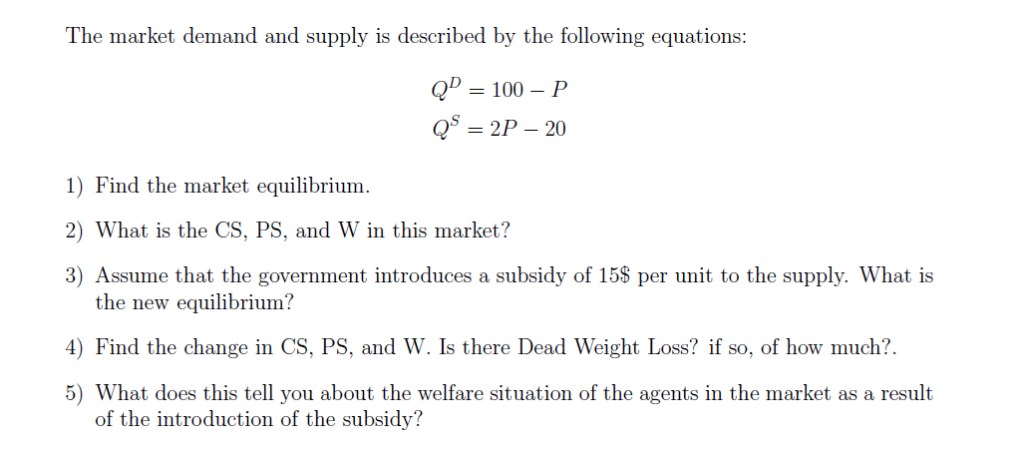The market demand and supply is described by the following equations: Q = 100 - P Q=2P - 20 1) Find the market equilibrium 2) What is the CS, PS, and W in this market? 3) Assume that the government introduces a subsidy of 15\$ per unit to the supply. What is the new equilibrium? 4) Find the change in CS, PS, and W. Is there Dead Weight Loss? if so, of how much?. 5) What does this tell you...

• ### Given the following market​ equations: ​ Supply: Qs ​ = -12   ​+ 3 p Demand: Qd=88-2p...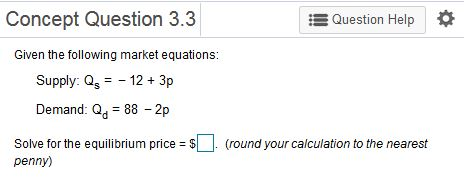Given the following market​ equations: ​ Supply: Qs ​ = -12   ​+ 3 p Demand: Qd=88-2p Solve for the equilibrium price​ = ​\$?? .   ​(round your calculation to the nearest penny​) Concept Question 3.3 Question Help Given the following market equations: Supply: Qs123p Demand: Q 88 -2p Solve for the equilibrium price-\$. (round your calculation to the nearest penny)

• ### Consider the following supply and demand equations, in the market for laddus: Supply:p= (1/5)q, Demand:p= 30−q....

Consider the following supply and demand equations, in the market for laddus: Supply:p= (1/5)q, Demand:p= 30−q. The government wants people to enjoy laddus at a reasonable price, and so enacts a price ceiling of ̄p= 1. What is the deadweight loss under this price ceiling?

• ### Suppose there exists a market for bicycles. The supply and the demand curves in this market...

Suppose there exists a market for bicycles. The supply and the demand curves in this market are given by the following equations where P is the price per bicycle measured in dollars and Q is the quantity of bicycles: Market Demand Curve: P = 1500 – 3Q Market Supply Curve: P = Q + 300. Given the above information and holding everything else constant, find the equilibrium price and quantity in this market.

• ### 3. Suppose the market for widgets can be described by the following equations: Demand: P= 10 - Q Supply: P=Q-4 where P...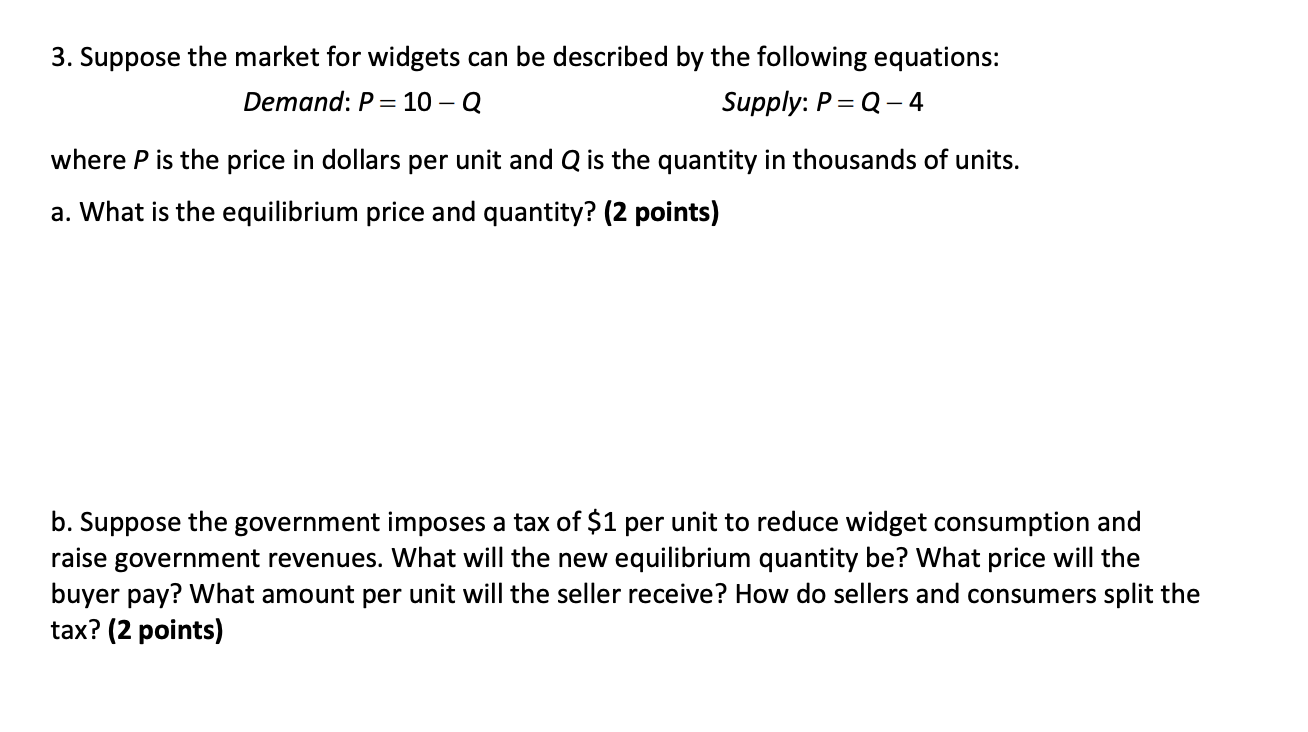3. Suppose the market for widgets can be described by the following equations: Demand: P= 10 - Q Supply: P=Q-4 where P is the price in dollars per unit and Q is the quantity in thousands of units. a. What is the equilibrium price and quantity? (2 points) b. Suppose the government imposes a tax of \$1 per unit to reduce widget consumption and raise government revenues. What will the new equilibrium quantity be? What price will the buyer pay?...

• ### #1 The market ties are competitive and demand is given by P=210-Q. If supply is given...

#1 The market ties are competitive and demand is given by P=210-Q. If supply is given by P=2Q, what will be the price in this market and what quantity? #2  Suppose that the administration requires all of its faculty and students to wear new ties every day. Draw a supply and demand graph of this situation indicating what will happen to the market price and quantity for the ties. Why does the price change in this market? #3 When P=5, quantity...

• ### Suppose that demand and supply are given by the following equations: P 85-18.Q P = 30+4...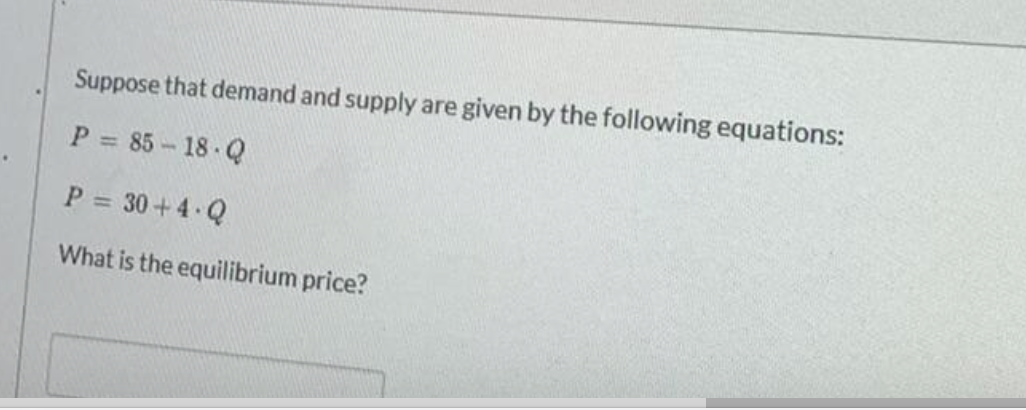Suppose that demand and supply are given by the following equations: P 85-18.Q P = 30+4 Q What is the equilibrium price?

Free Homework App